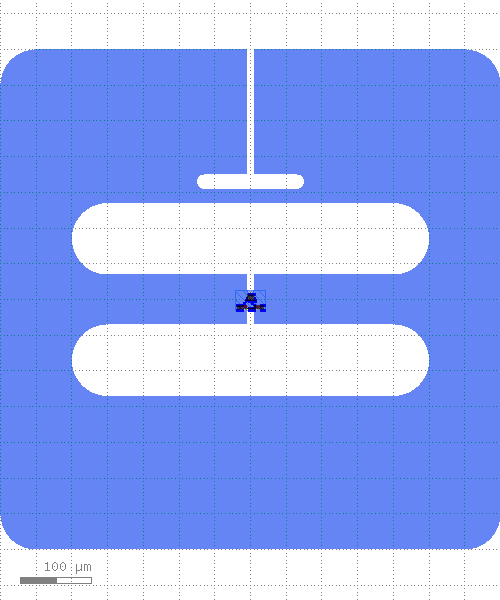A two-island qubit, consisting of two rounded rectangles shunted by a junction, with one capacitive coupler.

Contains a coupler on the north edge and two separate qubit islands in the center joined by a junction or SQUID loaded from another library. Refpoint for a readout line at the opening to the coupler and a modifiable refpoint for a driveline.

build()[source]

Child classes re-define this method to build the PCell.

classmethod get_sim_ports(simulation)[source]

List of RefpointToSimPort objects defining which refpoints should be turned to simulation ports for the given element class

Returns empty list if not implemented for Element subclass. When implementing this method, the best practice is for this method to have no “side effects”, that is all code contained within this method should only serve to derive the list of RefpointToSimPort objects and nothing else: no change in element’s geometry or parameter values.

Parameters
• cls – Element class, this is a class method

• simulation – Simulation object where a cell of this element class is placed. Use this argument if you need to decide certain arguments for RefpointToSimPort objects based on simulation’s parameters

Returns

List of RefpointToSimPort objects, empty list by default

PCell parameters:

• ground_gap (List) - Width, height of the ground gap (µm, µm), default=`[700, 700]`

• ground_gap_r (Double) - Ground gap rounding radius, default=`50`, unit=`μm`

• coupler_extent (List) - Width, height of the coupler (µm, µm), default=`[150, 20]`

• coupler_r (Double) - Coupler rounding radius, default=`10`, unit=`μm`

• coupler_a (Double) - Width of the coupler waveguide center conductor, default=`10`, unit=`μm`

• coupler_offset (Double) - Distance from first qubit island to coupler, default=`20`, unit=`μm`

• squid_offset (Double) - Offset between SQUID center and qubit center, default=`0`, unit=`μm`

• island1_extent (List) - Width, height of the first qubit island (µm, µm), default=`[500, 100]`

• island1_r (Double) - First qubit island rounding radius, default=`50`, unit=`μm`

• island2_extent (List) - Width, height of the second qubit island (µm, µm), default=`[500, 100]`

• island2_r (Double) - Second qubit island rounding radius, default=`50`, unit=`μm`

• drive_position (List) - Coordinate for the drive port (µm, µm), default=`[-450, 0]`

• island1_taper_width (Double) - First qubit island tapering width on the island side, default=`10`, unit=`µm`

• island1_taper_junction_width (Double) - First qubit island tapering width on the junction side, default=`10`, unit=`µm`

• island2_taper_width (Double) - Second qubit island tapering width on the island side, default=`10`, unit=`µm`

• island2_taper_junction_width (Double) - Second qubit island tapering width on the junction side, default=`10`, unit=`µm`

• island_island_gap (Double) - Island to island gap distance, default=`70`, unit=`µm`

• a (Double) - Width of center conductor, default=`10`, unit=`μm`

• b (Double) - Width of gap, default=`6`, unit=`μm`

• n (Int) - Number of points on turns, default=`64`

• r (Double) - Turn radius, default=`100`, unit=`μm`

• margin (Double) - Margin of the protection layer, default=`5`, unit=`μm`

• face_ids (List) - Chip face IDs list, default=`['1t1', '2b1', '1b1', '2t1']`

• display_name (String) - Name displayed in GUI (empty for default), default=

• protect_opposite_face (Boolean) - Add opposite face protection too, default=`False`

• junction_type (String) - Junction Type, default=`Manhattan`, choices=`['NoSquid', 'Manhattan', 'Manhattan Single Junction', 'Sim']`

• junction_width (Double) - Junction width (only used for code generated element), default=`0.02`, unit=`μm`

• junction_parameters (String) - Extra Junction Parameters, default=`{}`

• _junction_parameters (String) - Previous state of *_parameters, default=`{}`

• finger_overshoot (Double) - Length of fingers after the junction., default=`1.0`, unit=`μm`

• include_base_metal_gap (Boolean) - Include base metal gap layer, default=`True`

• include_base_metal_addition (Boolean) - Include base metal addition layer., default=`True`

• shadow_margin (Double) - Shadow layer margin near the the pads, default=`1.0`, unit=`μm`

• separate_junctions (Boolean) - Junctions to separate layer., default=`False`

• offset_compensation (Double) - Junction lead offset from junction width, default=`0`, unit=`μm`

• mirror_offset (Boolean) - Move the junction lead offset to the other lead, default=`False`

• finger_overlap (Double) - Length of fingers inside the pads., default=`0.2`, unit=`μm`

• height (Double) - Height of the junction element., default=`22.0`, unit=`μm`

• width (Double) - Width of the junction element., default=`22.0`, unit=`μm`

• pad_height (Double) - Height of the junction pad., default=`6.0`, unit=`μm`

• pad_width (Double) - Width of the junction pad., default=`12.0`, unit=`μm`

• pad_to_pad_separation (Double) - Pad separation., default=`6.0`, unit=`μm`

• x_offset (Double) - Horizontal junction offset, default=`0`, unit=`μm`

• loop_area (Double) - Loop area, default=`100`, unit=`μm^2`

• compact_geometry (Boolean) - Compact geometry for metal addition., default=`False`